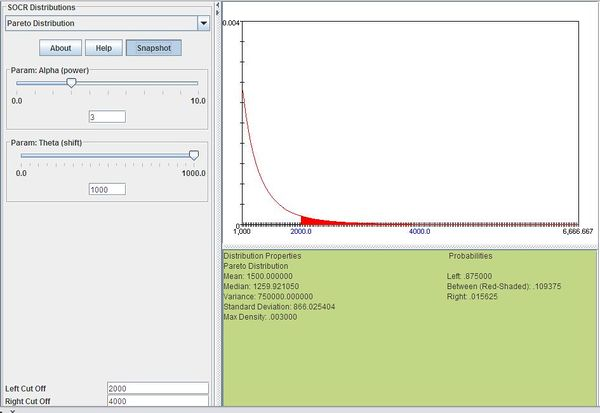# AP Statistics Curriculum 2007 Pareto

## General Advance-Placement (AP) Statistics Curriculum - Pareto Distribution

### Pareto Distribution

Definition: Pareto distribution is a skewed, heavy-tailed distribution that is sometimes used to model that distribution of incomes. The basis of the distribution is that a high proportion of a population has low income while only a few people have very high incomes.

Probability density function: For $$X\sim \operatorname{Pareto}(x_m,\alpha)\!$$, the Pareto probability density function is given by

$\frac{\alpha x_m^\alpha}{x^{\alpha+1}}$

where

• $$x_m$$ is the minimum possible value of X
• $$\alpha$$ is a positive parameter which determines the concentration of data towards the mode
• x is a random variable ($$x>x_m$$)

Cumulative density function: The Pareto cumulative distribution function is given by

$1-(\frac{x_m}{x})^\alpha$

where

• $$x_m$$ is the minimum possible value of X
• $$\alpha$$ is a positive parameter which determines the concentration of data towards the mode
• x is a random variable ($$x>x_m$$)

Moment generating function: The Pareto moment-generating function is

$M(t)=\alpha(-x_m t)^\alpha\Gamma(-\alpha,-x_m t)\!$

where

• $$\textstyle\Gamma(-\alpha,-x_m t)=\int_{-x_m t}^\infty t^{-\alpha-1}e^{-t}dt$$

Expectation: The expected value of Pareto distributed random variable x is

$E(X)=\frac{\alpha x_m}{\alpha-1}\mbox{ for }\alpha>1\!$

Variance: The Pareto variance is

$Var(X)=\frac{x_m^2 \alpha}{(\alpha-1)^2(\alpha-2)}\mbox{ for }\alpha>2\!$

### Applications

The Pareto distribution is sometimes expressed more simply as the “80-20 rule”, which describes a range of situations. In customer support, it means that 80% of problems come from 20% of customers. In economics, it means 80% of the wealth is controlled by 20% of the population. Examples of events that may be modeled by Pareto distribution include:

• The sizes of human settlements (few cities, many villages)
• The file size distribution of Internet traffic which uses the TCP protocol (few larger files, many smaller files)
• Hard disk drive error rates
• The values of oil reserves in oil fields (few large fields, many small fields)
• The length distribution in jobs assigned supercomputers (few large ones, many small ones)
• The standardized price returns on individual stocks
• The sizes of sand particles
• The sizes of meteorites
• The number of species per genus
• The areas burned in forest fires
• The severity of large casualty losses for certain businesses, such as general liability, commercial auto, and workers compensation

### Example

Suppose that the income of a certain population has a Pareto distribution with $$\alpha=3$$ and $$x_m=1000$$. Compute the proportion of the population with incomes between 2000 and 4000.

We can compute this as follows:

$P(2000\le X\le 4000)=\sum_{x=2000}^{4000}\frac{3\times 1000^3}{x^{3+1}}=0.109375$

The figure below shows this result using SOCR distributions"-----